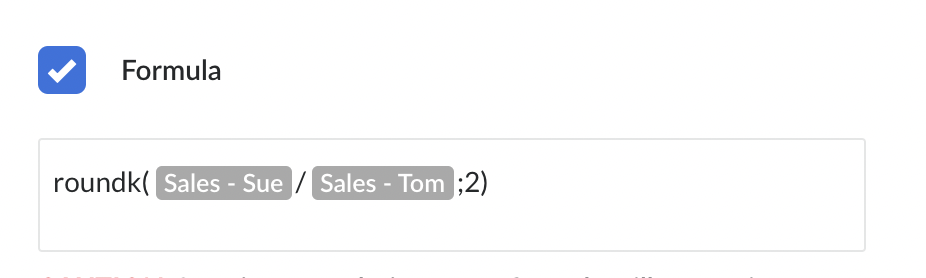# Formula - Rounding the result - RoundK

### Details:

Sometimes a formula will have an excess number of digits when there is only a need to show just a few numbers after the decimal. Use the ROUNDK ( A ; B ) expression to shorten the results of a formula. The ‘A’ portion is the formula, the ‘B’ is the maximum number of spaces a number can display after the decimal point.

### Steps:

1. Create a new measurable by clicking in the + sign in the top right corner of the Scorecard tile or in the “edit meeting” section.
2. Fill in the name of the measurable, select your goal, click on the Formula checkbox on the right side of the screen.
3. Type in the measurables names on which you want to base your calculations off. (Eg. Measurable1/Measurable2 or Measurable1/0.2)
4. Type in “RoundK” at the beginning of the line.
5. Open parenthesis between “RoundK” and the mathematical operation.
6. Type in a semicolon at the end of the mathematical operation/equation and the number of decimal places you want to round your results.
7. Close parenthesis at the end of the formula.